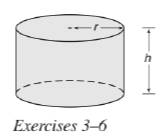Chapter 9.3, Problem 6EElementary Geometry For College St...

7th Edition
Alexander + 2 others
ISBN: 9781337614085

Solutions

Chapter
SectionElementary Geometry For College St...

7th Edition
Alexander + 2 others
ISBN: 9781337614085
Textbook ProblemSuppose that r = 12 cm and h = 15 cm in the right circular cylinder. Find the exact and approximatea) lateral area.b) total area.c) volume.

To determine

(a)

To calculate: The exact and approximate lateral area of the right circular cylinder.

Explanation

Given:

For a right circular cylinder r=12 cm and h=15 cm.

Formula used:

The lateral area of the right circular cylinder is L=2πrh.

Calculation:

Substitute the known values in the formula, we get

L=2πrh

To determine

(b)

To calculate: The exact and approximate total area of the right circular cylinder.

To determine

(c)

To calculate: The exact and approximate volume of the right circular cylinder.

Still sussing out bartleby?

Check out a sample textbook solution.

See a sample solution

The Solution to Your Study Problems

Bartleby provides explanations to thousands of textbook problems written by our experts, many with advanced degrees!

Get Started

Find the remaining trigonometric ratios. 34. csc=43,322

Single Variable Calculus: Early Transcendentals, Volume I

For the following set of scores, find the value of each expression: a. X b. (X)2 c. X2 d. (X +3)

Essentials of Statistics for The Behavioral Sciences (MindTap Course List)

Simplify the expressions in Exercises 97106. 41/147/2

Finite Mathematics and Applied Calculus (MindTap Course List)

Evaluate the integral. 01(u+2)(u3)du

Single Variable Calculus: Early Transcendentals